Next: 3.2.5 The Fourier Transform Up: 3.2 Measuring the Internal Previous: 3.2.3 The Relaxation Function

## 3.2.4 Four-Counter Geometry and the Complex Polarization

Now consider the complete set of four positron counters in Fig. 3.1. Ignoring geometric misalignments and differences in counter efficiency, the x-component of the muon polarization Px(t) [monitored by the L and R counters] differs from the y-component of the muon polarization Py(t) [monitored by the U and D counters] by a phase of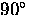. The two components of the muon polarization can be combined to form a complex'' polarization function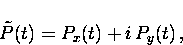(25)
where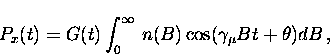(26)
and

The complex asymmetry for the four-counter setup is defined as

where Ax(t) and Ay(t) are the real and imaginary parts of the complex asymmetry, respectively. The number of counts per second in the ith counter (i = L, R, U or D) is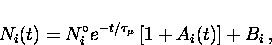(27)
where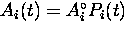is the asymmetry function for the ith raw histogram. Rearranging Eq. (3.29) gives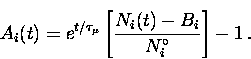(28)
In terms of the individual counters, the real asymmetry Ax(t) and the imaginary asymmetry Ay(t) are

In this thesis the real and imaginary parts of the asymmetry were fit simultaneously, assuming a phase difference ofbetween them.Next: 3.2.5 The Fourier Transform Up: 3.2 Measuring the Internal Previous: 3.2.3 The Relaxation Function